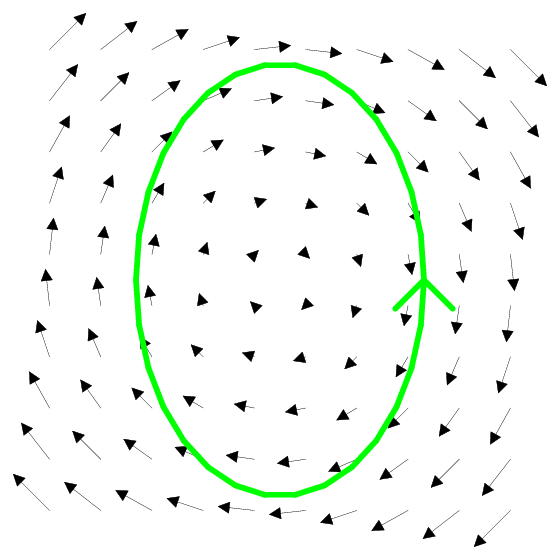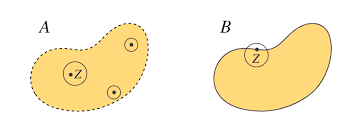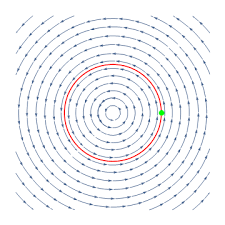# Topology & Calculus

If one is familiar with line integrals from calculus, one may have encountered vocabulary used in theorems to describe regions such as open and closed, connected , and simply connected. These all have relatively approachable and easy to understand definitions when considering closed line integrals in $$\mathbb{R}^2$$ with interesting topological definitions for other spaces. As any calculus 3 student could tell you, the line integrals for which these definitions are used are force integrals, more specifically the integral along a path of Fᐧdr. A closed line integral is then one for which the path is a complete loop, that is for the curve from $$t = a$$ to $$t = b, f(a) = f(b)$$. Below is an example of a closed line integral in a force field.Before translating calculus vocabulary to topological ones, it is necessary to build a foundation in ideas of topology. A given topology is a structure which is defined on an underlying set; consider that $$\mathbb{R}^2$$ is the underlying set when discussing the standard, or Euclidean, topology. The topology on the set contains a collection of open sets in which the entire set, the empty set, and unions and intersections of open sets are all open in the topology. The structure of the topology is what dictates what constitutes an open set. The Euclidean topology is the natural topology on anything of the form $$\mathbb{R}^n$$, in which open sets are balls around a point where the distance from a point in that ball to the center point is less than some value. A good check for understanding is to verify that open intervals on the real number line are open sets in $$\mathbb{R}$$. Finally, a closed set is the complement of an open set; a more visually intuitive definition is that a closed set is a set that contains its limit points, such as closed intervals containing the bounds.Now that we can discuss open and closed regions, such as the closed region bounded by a closed line integral, we are ready to analyze these regions. A space, or region, is said to be connected if it cannot be represented as the union of disjoint, non-empty, open subsets. For regions in $$\mathbb{R}^2$$, the definition boils down to the region is not clearly numerous distinct regions, as seen below.In $$\mathbb{R}^2$$, a region is path connected if there exists at least one path between any two points in the region. Topologically, a space is path connected if there is a continuous function, $$f$$, from [0,1] to the space where $$f(0)$$ is the start point and $$f(1)$$ is the end point. The notion of a continuous function is the generalization of drawing a continous path in $$\mathbb{R}^2$$.Finally, a simply connected region is one where any path between two points can be continuously mapped into any other path between those two points. This can be visualized in $$\mathbb{R}^2$$ by a string connected at two points and deforming the path of the string into other paths by only moving the string without any cutting or removing end connections, keeping the string within the space. In $$\mathbb{R}^2$$, any region with holes is not simply connected because the path cannot be continuously mapped over that hole because the path would have to “jump” from one side to the other.The restrictions of the theorems exist for the “.1%” of cases of vector fields that have strange behavior without these rules. The vortex vector field, $=$, is an example of when these restrictions are crucial to producing the right answer. The problem with that vector field is that there is a discontinuity at the origin, meaning that regions that should enclose the origin don’t act the same way as they would in other vector fields. This is because the field exists on the punctured plane, $$\mathbb{R}^2$$ without the origin, which is a different topology than $$\mathbb{R}^2$$ in which the theorems apply. As the reader can, and should, check, the region enclosed by the unit circle in the vortex vector field is a connected, closed, path connected region that is not simply connected. The reader is now encouraged to explore the connectedness of closed line integrals enclosing the origin in other vector fields on the punctured plane.Contributor: Joseph Brewster